# Gross Domestic Product (GDP)

• Updated on

## What is Gross Domestic Product (GDP)?

GDP refers to the total market value of goods and services produced by a country over a specific period. It is one of the most important economic indicators and is a commonly used benchmark against which economic well-being is measured.

In oversimplified terms, GDP is the quantity of goods and services produced by a country multiplied with their respective prices, summed over different sectors. However, there are various methods for categorising domestic produce into different sectors. This complicates the GDP calculation further away from its basic ‘quantity multiplied by price’ definition.

It is important to avoid the problem of double-counting while calculating GDP. Double counting refers to adding the input twice in the calculation, once as a good produced and the second time as the input cost in the final good. The problem of double-counting is removed when only the final product is included in the calculation and not the intermediary inputs.

GDP can be calculated at Factor Cost (called GDP at FC) or at Market Price (called GDP at MP). GDP at FC refers to the quantity of goods and services multiplied with their factor cost and summed over sectors. Factor cost refers to the cost of factor inputs, namely, rent, wage, interest and profits.

Market prices are derived by adding indirect taxes and subtracting subsidies to GDP at FC. The GDP figures that are often referred to as the ‘size’ of an economy are usually GDP calculated at Market Price.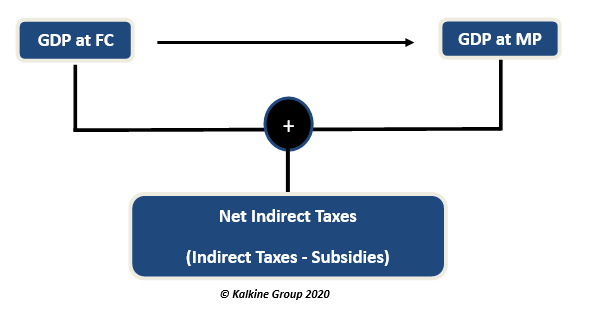## How is GDP calculated?

There are three approaches that are used to calculate GDP, namely Expenditure Approach, the Income Approach, and the Output Approach. GDP calculated from either of these would give the same results.

1. Expenditure Approach: As the name suggests, the expenditure approach uses the total spending incurred by households, businesses, and the government. This method includes 4 major components, namely, Consumption, Investment, Government Expenditure and Net Exports.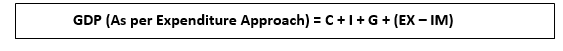Consumption expenditure (C) refers to the expenditure done by consumers on everyday items as well as other household expenses,

Government Expenditure (G) refers to spending by the Government on domestic projects, policies, funds, etc,

Net Exports are calculated by subtracting Imports (IM) from Exports (EX).

All these components are from the ‘spending side’ of a transaction.

1. Income Approach: This method involves adding the income generated by the factors of production. This is kind of like a middle ground between the other two approaches.

The producers of domestic goods and services are the factors of production and the income generated by them is known as the factor income. Using Income Approach, we can achieve Net National Income (NNI) which is different from GDP.

Here, the income approach formula for NNI is,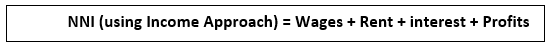Now we can find out the GDP at FC using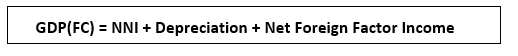And finally, GDP at MP using,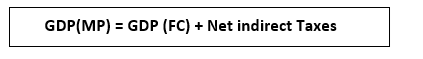1. Output Approach: Here only the real GDP or the real output levels (and not nominal) are considered. This method can be understood as the approach taken from the opposite end of the expenditure approach. It includes the production costs incurred in producing the final good. Here, once again the cost of intermediary inputs is not included to avoid the problem of double counting.

## What are the different types of GDP?

GDP has two broad types: nominal GDP and real GDP. Nominal GDP includes the prices of the corresponding goods and services and is not adjusted for inflation. Whereas real GDP refers to the GDP adjusted for inflation and it gives a more accurate representation of the production levels in the economy. This allows for comparisons between different time periods. GDP deflator can be used to remove the effect of inflation on the economy and move from nominal to real GDP.

Real GDP is a powerful tool to judge the health of an economy. Rate of change of real GDP provides further depth into the strength of the economy. If real GDP is increasing (or Rate of growth of real GDP is positive) then it means the economy is expanding. Besides, employment is likely to rise, and it may also encourage foreign investments into the country. Most developing countries see a high rate of growth of GDP.

On the other hand, a contracting real GDP (or falling Rate of growth of GDP) could mean that there is not enough production happening in the markets. If the rate of growth of GDP is very low, it might indicate the possibility of saturated markets. Highly developed nations usually experience a low rate of growth of GDP, which is typically lower than that of developing countries.

## What are the risks associated with GDP as an economic indicator?

GDP as an economic indicator does not hold information about the entire economy. There are certain aspects that cannot be revealed through GDP figures. The socio-economic conditions, literacy levels, environmental damages done by a nation and the state of living conditions of a country are just some of the aspects that cannot be incorporated into GDP calculation.

It is quite possible that countries with high levels of GDP are achieving the same by harming the environment, while countries with low GDP rates are highly developed nations with good living standards and better literacy levels.

There have been various other indicators that capture these socio-economic aspects of a nation. One major indicator is the Human Development Index, which incorporates components ranging from literacy rates, to population and mortality rates. This is a more wholistic approach than GDP rate as it is more inclusive. There are various such indices adopted by countries which try to create the vision that overall strength of a nation is represented through a lot more than the rate at which it produces its output.